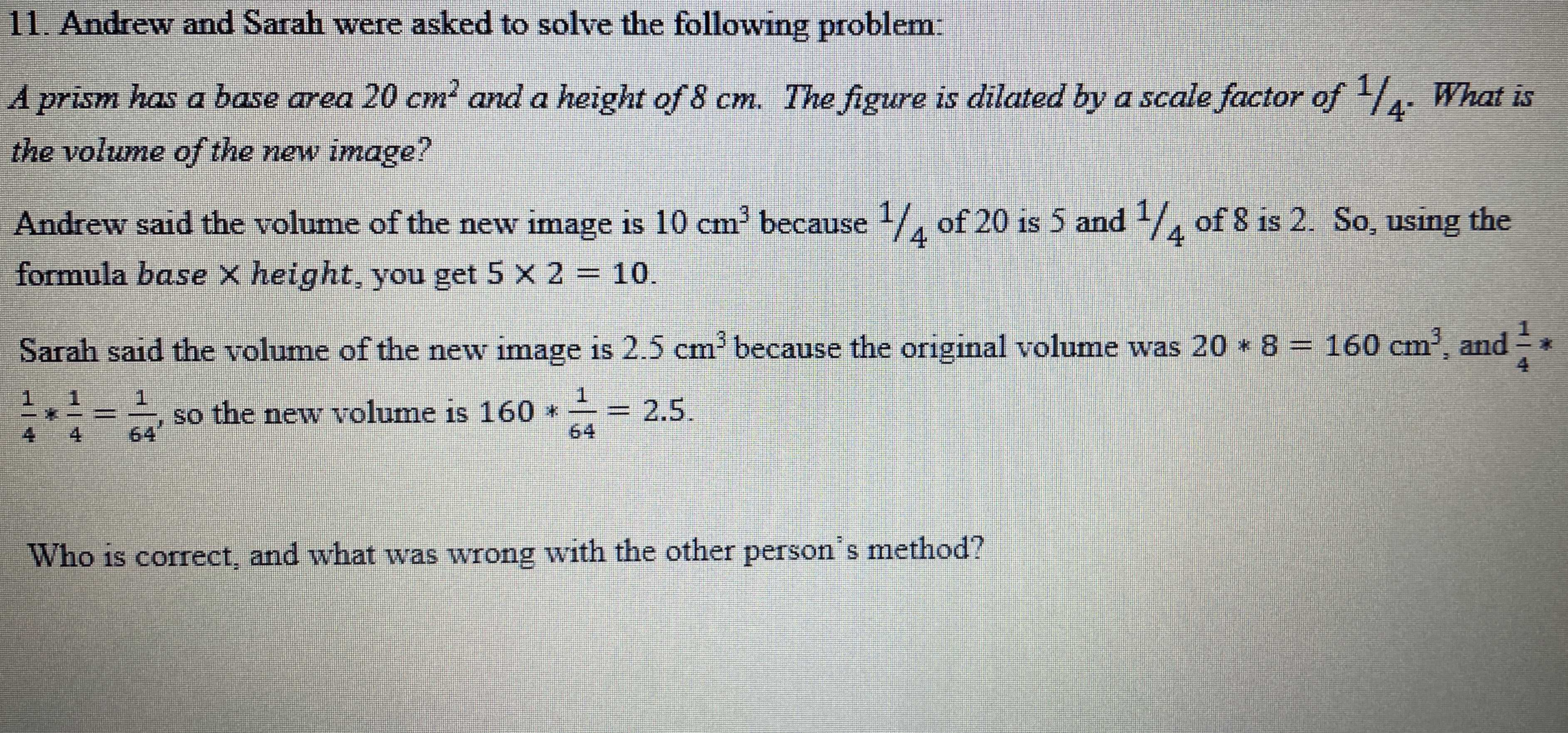### ¿Todavía tienes preguntas de matemáticas?

Pregunte a nuestros tutores expertos
Algebra
Pregunta11. Andrew and Sarah were asked to solve the following problem: A prism has a base area $$20 cm ^ { 2 }$$ and a height of $$8 cm$$ . The figure is dilated by a scale factor of $$1 / 4$$ . What is the volume of the new image? Andrew said the volume of the new image is $$10 cm ^ { 3 }$$ because $$1 / 4$$ of $$20$$ is $$5$$ and $$1 / 4$$ of $$8$$ is $$2$$ . So, using the formula base $$\times$$ height, you get $$5 \times 2 = 10$$ . Sarah said the volume of the new image is $$2.5 cm ^ { 3 }$$ because the original volume was $$20 * 8 = 160 cm ^ { 3 }$$ , and $$\frac { 1 } { 4 } *$$

$$\frac { 1 } { 4 } * \frac { 1 } { 4 } = \frac { 1 } { 64 }$$ , so the new volume is $$160 * \frac { 1 } { 64 } = 2.5$$ .

Who is correct, and what was wrong with the other person's method?